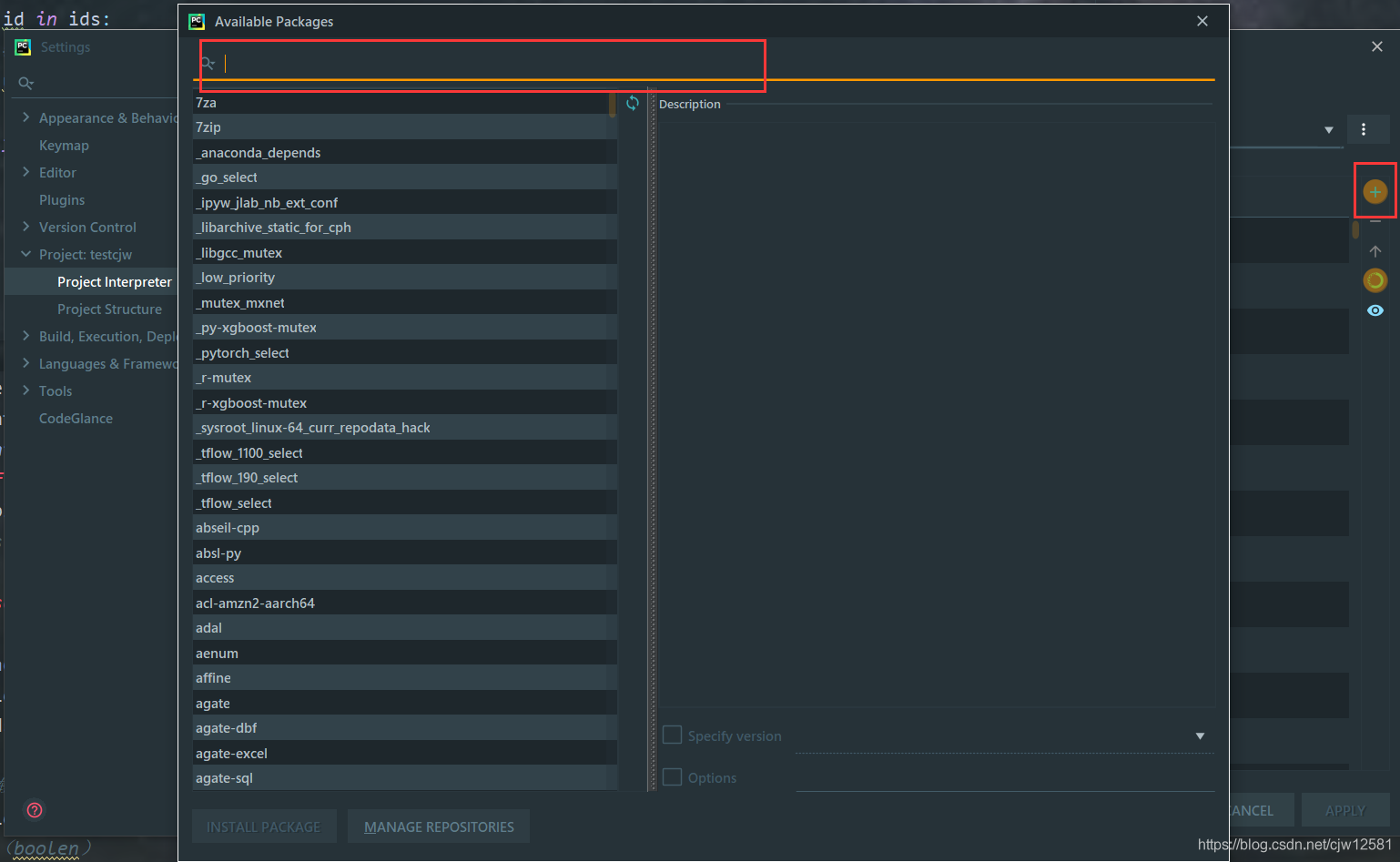# Matplotlib下载、安装、简单使用# Matplotlib

Matplotlib是一个Python 2D绘图库，它以多种硬拷贝格式和跨平台的交互式环境生成出版物质量的图形。它可与 NumPy 一起使用，提供了一种有效的 MatLab 开源替代方案，也可用于Python脚本，Python和IPython (opens new window)Shell、Jupyter (opens new window)笔记本，Web应用程序服务器和 图形用户界面工具包.(PyQt、wxPython、PyGObject、PyGTK、PySide、Tkinter)。
Matplotlib：中文文档官方网站
Matplotlib 尝试使容易的事情变得更容易，使困难的事情变得可能。 只需几行代码就可以生成图表、直方图、功率谱、条形图、误差图、散点图等。为了简单绘图，该 pyplot 模块提供了类似于MATLAB的界面，尤其是与Python结合使用时。 对于高级用户，可以通过面向对象的界面或MATLAB用户熟悉的一组功能来完全控制线型，字体属性，轴属性等。

## 下载安装（windows）

1.命令行安装

``````pip install matplotlib
``````

2.在PyCharm中安装# 使用

``````import numpy as np
from matplotlib import pyplot as plt

x = np.arange(1,11)
y =  2  * x +  5
plt.title("Matplotlib demo")
plt.xlabel("x axis caption")
plt.ylabel("y axis caption")
plt.plot(x,y)
plt.show()
``````

END